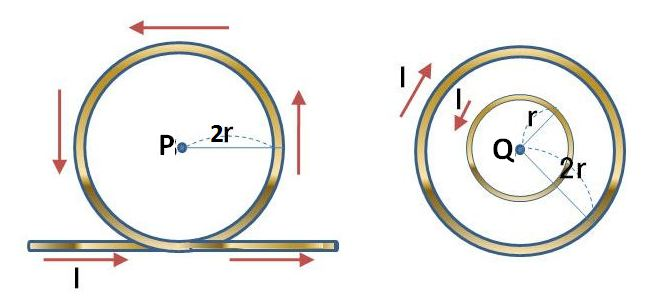# Magnetic field from loop currentThe above left diagram shows a circular current with radius $2r$ and current intensity $I,$ flowing in the direction of the arrows. The above right diagram shows two concentric circular currents with radii $r$ and $2r,$ respectively, and the same current intensity $I,$ flowing in the direction of their respective arrows. Points P and Q are the centers of their respective circular currents. If the magnetic field intensity at point P is $B,$ then what is the magnetic field intensity at point Q?

×

Problem Loading...

Note Loading...

Set Loading...